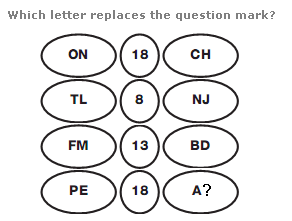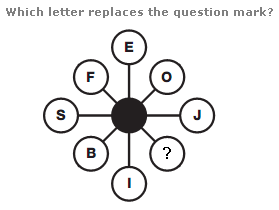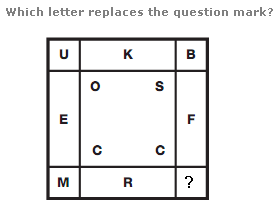# Puzzles - Missing letters puzzles

### Exercise :: Missing letters puzzlesAnswer : B Explanation : In each row, the value in the centre equals the difference between the sum of the numerical values of the pair of letters to the left and the pair of letters to the right.Answer : K Explanation : The sum of the letters in the three adjacent circles on each side of the diagram is always 30.Answer : D Explanation : The diagram contains 3 sets of 4 letters - one set of outer corner letters, one set of inner corner letters, and one set of letters on each side of the square. The numerical values of the letters in each set add up to 40.